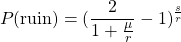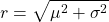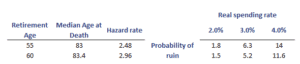# Probability of ruin

The probability of ruin equals the probability that a portfolio will reach a value of zero. It is used to inform investors about the probability that the portfolio will not meet the objectives. Typically, ruin risk is used in the context of retirement planning, where the risk of ruin is based on differing start dates for retirement and different distribution percentages. By delaying retirement or lowering the distribution percentage, it is possible to lower the risk of ruin considerably.

On this page, we discuss the probability of ruin formula and illustrate the approach using a ruin risk table.

## Definition ruin probability

The ruin risk equals the likelihood that a portfolio will reach the value zero. In that case, the person owning the portfolio will have no assets left and is thus ruined. Everyone, of course, wants to avoid being ruined. At the same time, ensuring the portfolio grows over time requires investing the money which entails risk. Thus, it is impossible to invest and simultaneously have a ruin risk equal to zero. In practice, the likelihood of running out of funds is presented using a ruin probability table

## Formula

The formula is the following:where r equalswhere is mu is the mean and sigma is the standard deviation of returns. s is the starting value.

## Ruin probability table example

Let’s have a look at a ruin probability example. The following table is an example. It indicates that if the person were to retire at 55 and would reach the age of 83, there is a 14% likelihood of running out of assets in the case of a 4% real spending rate. If the person’s spending rate only grows at 2%, the probability of ruin is only 1.8%## Summary

We discussed ruin risk, a useful concept in the context of retirement planning. It is a convenient way to help investors grasp the likelihood of the portfolio reaching a value of zero before death.